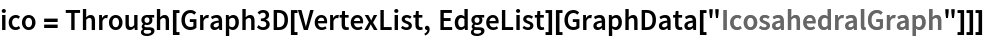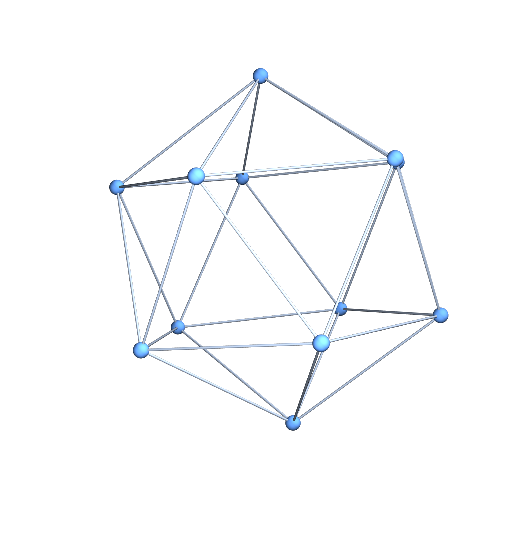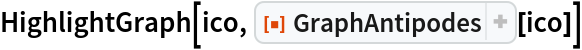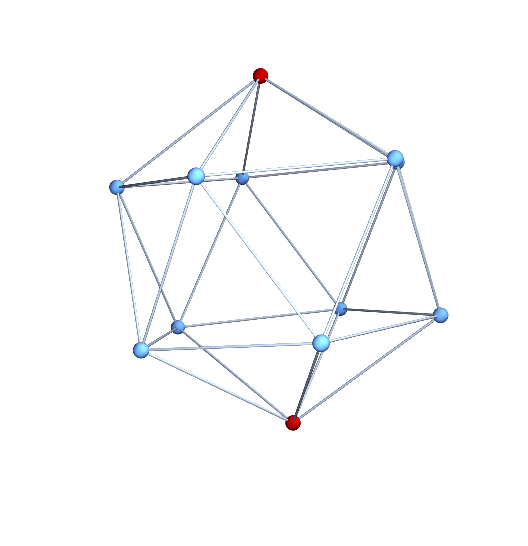Function Repository Resource:

# GraphAntipodes

Find a pair of vertices that are a maximum distance apart on a graph

Contributed by: Stephen Wolfram and Jan Mangaldan
 ResourceFunction["GraphAntipodes"][g] gives a pair of vertices of graph g that have the greatest distance from each other.

## Examples

### Basic Examples (2)

Generate an icosahedral graph:

 In:=Out=Highlight a pair of vertices that have the greatest graph distance from each other:

 In:=Out=## Version History

• 1.0.0 – 17 March 2020